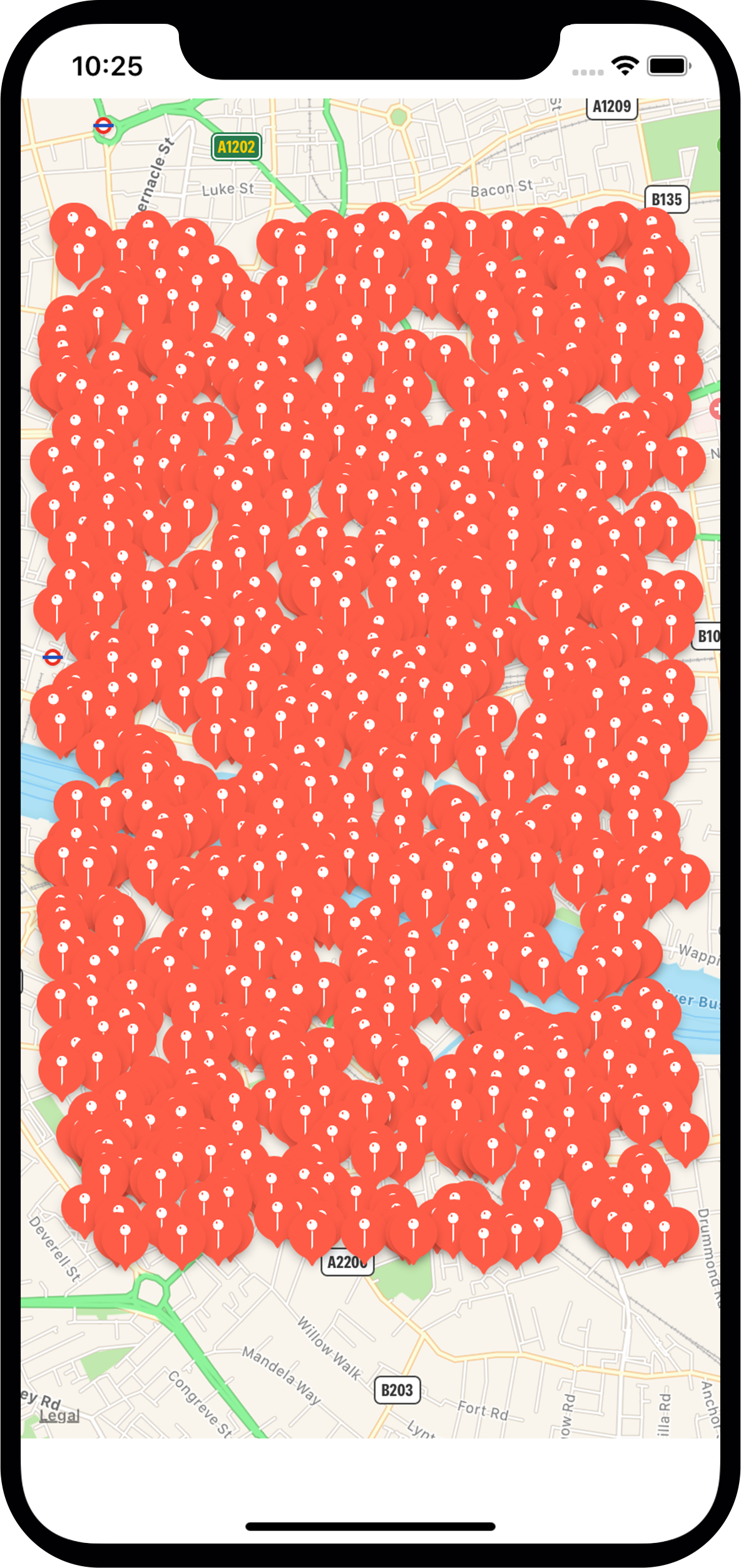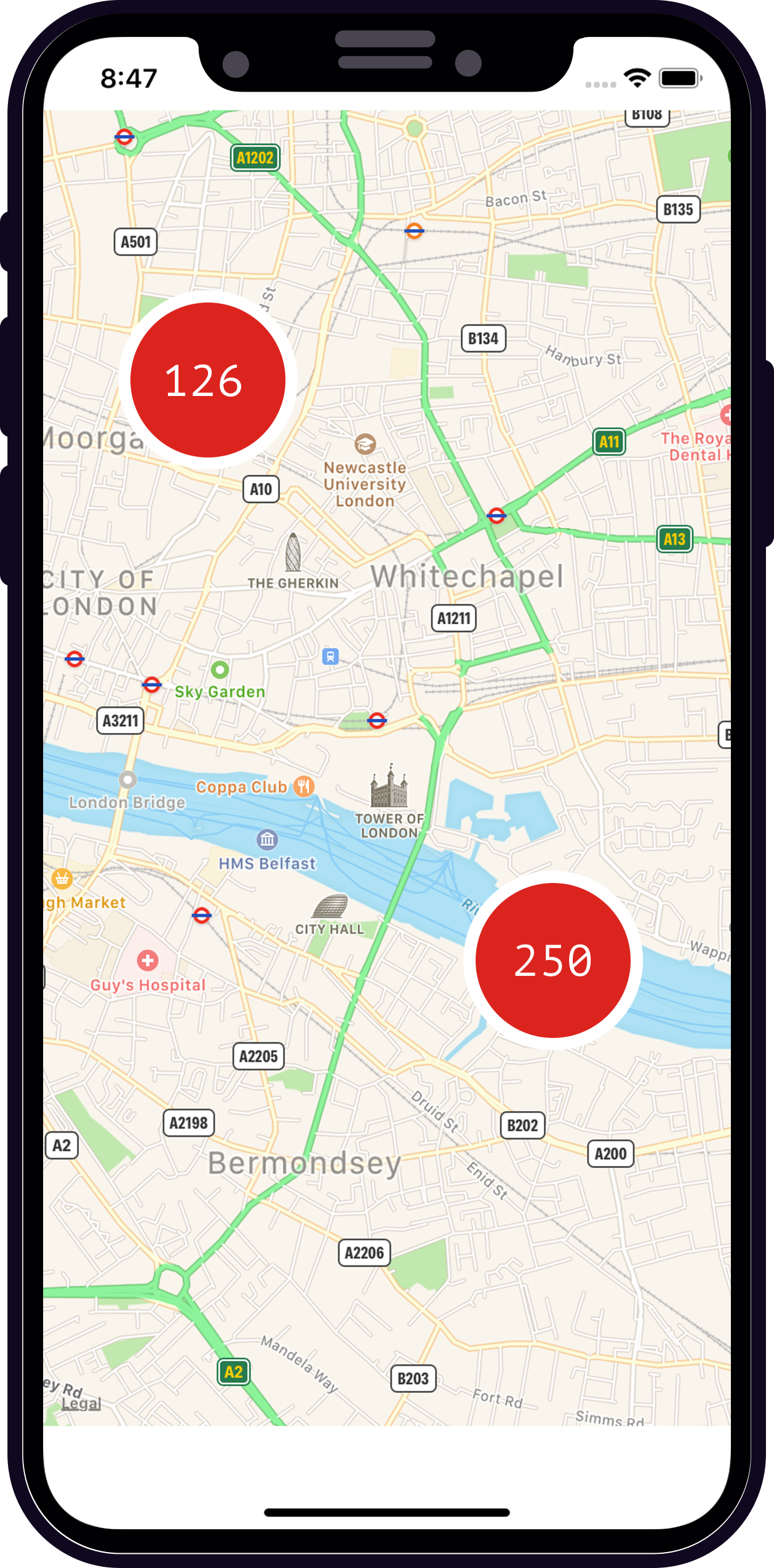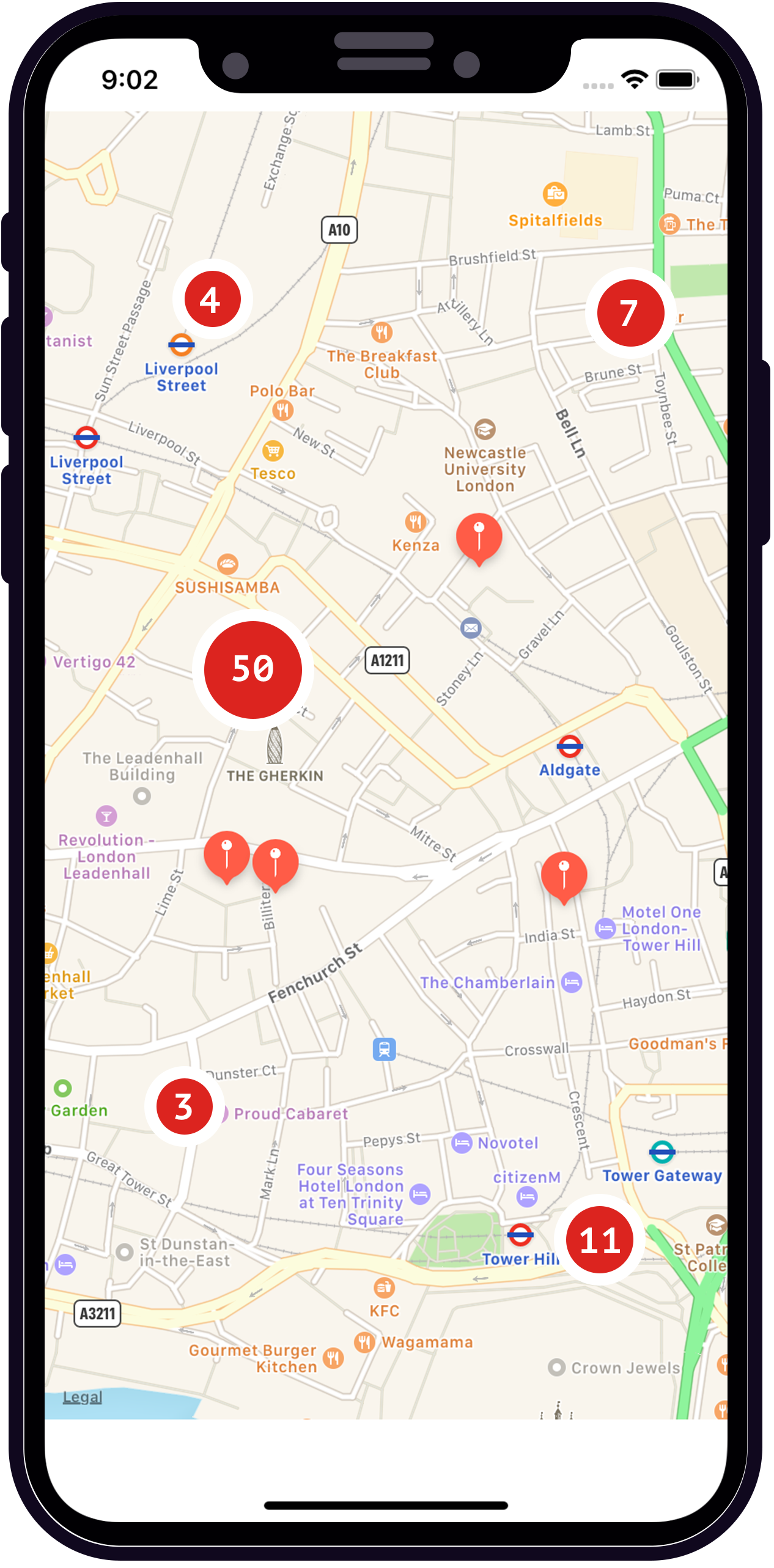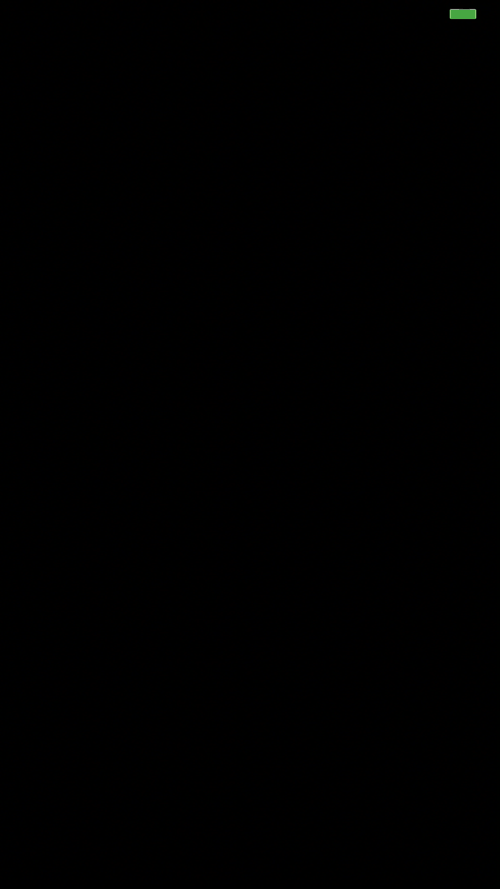Pablo Garcia

Apps for iPhone, iPad, Apple TV and Apple Watch

# Quadtree and Map Clustering - I

Jan 23, 2019

Nowadays, lot of apps use maps to show some information, great apps like Starbucks Near Me, Luas Dublin etc. But, what happen if there are a lot of annotations to show? probably we'd face up something like this.Really annoying, isn't it? This map is crowded and it's very difficult to get significant information from it.

One way to solve this problem is by grouping points that are at a certain distance from each other on the screen. At a low zoom levels (like the image shown above) we'll show a few groups of points and at a high zoom levels more groups.This technique is called Clustering. Clustering is the task of grouping a set of objects in such a way that objects in the same group (called a cluster) are more similar (in some sense, i.e. distance) to each other than to those in other groups (clusters).

Basically this is a search problem, we'll need to find all the points which lie in different regions of the map to create groups of points. QuadTree is a data structure that can efficiently find all locations contained in a specific region. It's built by recursively subdividing a two-dimensional space into smaller regions.

Each node of the QuadTree will have a list of points (in that node), the capacity and a bounding box. When we insert a new point in the QuadTree, first we check if the node's bounding box contains the coordinates of the new point, if so, we check if the node is full. If not, we can insert the point, but if the node is full, we have to divide it in four small nodes and try to insert the point in each of this child nodes.

``````

static let capacity = 4

var points = [CGPoint]()
var boundingBox = CGRect()
var isDivided = false

init(boundingBox: CGRect) {
self.boundingBox = boundingBox
}

public func insertPoint(newPoint: CGPoint) {

guard self.boundingBox.contains(newPoint) else {
return
}
if points.count < QuadTree.capacity && northWest == nil {
points.append(newPoint)
} else {
if northWest == nil {
self.subdivide()
}
northWest?.insertPoint(newPoint: newPoint)
northEast?.insertPoint(newPoint: newPoint)
southWest?.insertPoint(newPoint: newPoint)
southEast?.insertPoint(newPoint: newPoint)
}
}
private func subdivide() {
self.isDivided = true
let width = self.boundingBox.width/2
let height = self.boundingBox.height/2
let size = CGSize(width: width-offset, height: height-offset)

self.northWest = QuadTree(boundingBox: CGRect(origin: CGPoint(x: self.boundingBox.origin.x,
y: self.boundingBox.origin.y),
size: size))
self.northEast = QuadTree(boundingBox: CGRect(origin: CGPoint(x: self.boundingBox.origin.x+width,
y: self.boundingBox.origin.y),
size: size))
self.southWest = QuadTree(boundingBox: CGRect(origin: CGPoint(x: self.boundingBox.origin.x,
y: self.boundingBox.origin.y+height),
size: size))
self.southEast = QuadTree(boundingBox: CGRect(origin: CGPoint(x: self.boundingBox.origin.x+width,
y: self.boundingBox.origin.y+height),
size: size))
}
}

``````

Here you can see my QuadTree in action.See you in Quadtree and Map Clustering - II